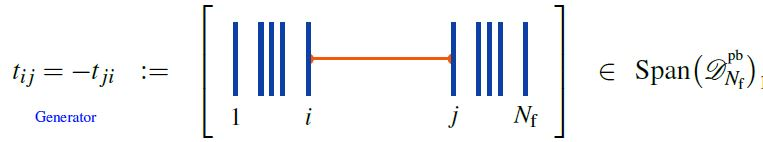# nLab Kontsevich integral

The Kontsevich Integral

### Context

#### Knot theory

knot theory

Examples/classes:

Types

knot invariants

Related concepts:

category: knot theory

# The Kontsevich Integral

## Idea

The Kontsevich integral is the Dyson formula for the parallel transport or holonomy of the Knizhnik-Zamolodchikov connection on ordered configuration spaces of points in the plane. As such it is a (universal) Vassiliev invariant of braids and, with due care, of knots and links, essentially coinciding with the Wilson loop observable of perturbatively quantized Chern-Simons theory.

The Kontsevich integral generalises the Gauss integral formula? which computes the linking number of two embedded circles via integration.

## Definition

### On braids

For the Definition of the Knizhnik-Zamolodchikov connection we need the following notation:

1. configuration spaces of points

For $N_{\mathrm{f}} \in \mathbb{N}$ write

(1)$\underset{{}^{\{1,\cdots,N_{\mathrm{f}}\}}}{Conf}(\mathbb{R}^2) \;\coloneqq\; (\mathbb{R}^2)^n \backslash FatDiagonal$

for the ordered configuration space of n points in the plane, regarded as a smooth manifold.

Identifying the plane with the complex plane $\mathbb{C}$, we have canonical holomorphic coordinate functions

(2)$(z_1, \cdots, z_{N_{\mathrm{f}}}) \;\colon\; \underset{{}^{\{1,\cdots,n\}}}{Conf}(\mathbb{R}^2) \longrightarrow \mathbb{C}^{N_{\mathrm{f}}} \,.$
2. horizontal chord diagrams

(3)$\mathcal{A}^{{}^{pb}}_{N_{\mathrm{f}}} \;\coloneqq\; Span\big(\mathcal{D}^{{}^{pb}}_{N_{\mathrm{f}}}\big)/4T$

for the quotient vector space of the linear span of horizontal chord diagrams on $n$ strands by the 4T relations (infinitesimal braid relations), regarded as an associative algebra under concatenation of strands (here).

###### Definition

(Knizhnik-Zamolodchikov form)

(4)$\omega_{KZ} \;\in\; \Omega \big( \underset{{}^{\{1,\cdots,N_{\mathrm{f}}\}}}{Conf}(\mathbb{C}) \,, \mathcal{A}^{{}^{pb}}_{N_{\mathrm{f}}} \big)$

given in the canonical coordinates (2) by:

(5)$\omega_{KZ} \;\coloneqq\; \underset{ i \lt j \in \{1, \cdots, n\} }{\sum} d_{dR} log\big( z_i - z_j \big) \otimes t_{i j} \,,$

whereis the horizontal chord diagram with exactly one chord, which stretches between the $i$th and the $j$th strand.

Regarded as a connection form for a connection on a vector bundle, this defines the universal Knizhnik-Zamolodchikov connection $\nabla_{KZ}$, with covariant derivative

$\nabla \phi \;\coloneqq\; d \phi + \omega_{KV} \wedge \phi$

for any smooth function

$\phi \;\colon\; \underset{{}^{\{1,\cdots,N_{\mathrm{f}}\}}}{Conf}(\mathbb{C}) \longrightarrow \mathcal{A}^{{}^{pb}}_{N_{\mathrm{f}}} Mod$

with values in modules over the algebra of horizontal chord diagrams modulo 4T relations.

The condition of covariant constancy

$\nabla_{KZ} \phi \;=\; 0$

is called the Knizhnik-Zamolodchikov equation.

Finally, given a metric Lie algebra $\mathfrak{g}$ and a tuple of Lie algebra representations

$( V_1, \cdots, V_{N_{\mathrm{f}}} ) \;\in\; (\mathfrak{g} Rep_{/\sim})^{N_{\mathrm{f}}} \,,$

the corresponding endomorphism-valued Lie algebra weight system

$w_{V} \;\colon\; \mathcal{A}^{{}^{pf}}_{N_{\mathrm{f}}} \longrightarrow End_{\mathfrak{g}}\big( V_1 \otimes \cdots V_{N_{\mathrm{f}}} \big)$

turns the universal Knizhnik-Zamolodchikov form (4) into a endomorphism ring-valued differential form

(6)$\omega_{KZ} \;\coloneqq\; \underset{ i \lt j \in \{1, \cdots, n\} }{\sum} d_{dR} log\big( z_i - z_j \big) \otimes w_V(t_{i j}) \;\in\; \Omega \big( \underset{{}^{\{1,\cdots,N_{\mathrm{f}}\}}}{Conf}(\mathbb{C}) \,, End\big(V_1 \otimes \cdot V_{N_{\mathrm{f}}} \big) \big) \,.$

The universal formulation (4) is highlighted for instance in Bat-Natan 95, Section 4.2, Lescop 00, p. 7. Most authors state the version after evaluation in a Lie algebra weight system, e.g. Kohno 14, Section 5.

###### Proposition

(Knizhnik-Zamolodchikov connection is flat)

The Knizhnik-Zamolodchikov connection $\omega_{ZK}$ (Def. ) is flat:

$d \omega_{ZK} + \omega_{ZK} \wedge \omega_{ZK} \;=\; 0 \,.$
###### Proposition

(Kontsevich integral for braids)

The Dyson formula for the holonomy of the Knizhnik-Zamolodchikov connection (Def. ) is called the Kontsevich integral on braids.

### On knots

###### Definition

Let $K$ be a strict Morse knot?. Let $\widehat{\mathcal{A}}$ be the graded completion? of the algebra of chord diagrams with $1$-term relations. The Kontsevich integral of $K$ is given by:

$Z(K) \;=\; \sum_{m = 0}^\infty \frac{1}{(2 \pi i)^m} \int_{t_{\min} \lt t_m \lt \cdots \lt t_1 \lt t_{\max} \over t_j\; \text{non-critical}} \sum_{P = \{(z_j,z_j')\}} (-1)^{\downarrow P} D_p \bigwedge_{j=1}^m \frac{d z_j - d z_j'}{z_j - z_j'}$

In this definition:

• $t_{\min}$ and $t_{\max}$ are the minimum and maximum of the $t$-coordinate in the Morse knot? $K$.
• The integration is over the points in the simplex of $m$ points in the interval $[t_{\min},t_{\max}]$ where no coordinate is critical on $K$.
• Upon removing the critical values (note: values not points, so we remove a point if it is on the same level as a critical point), the knot decomposes into a set of arcs which can be parametrised by height. Each arc therefore defines a function $z \colon I \to \mathbb{C}$ where $I$ is the corresponding interval of height values. In fact, $I$ must be the open interval between two successive critical values of the height function. For a particular such interval, there must be an even number of arcs with that domain. Given a point in the simplex (with no critical values), each coordinate in that point lies in an interval between critical values, and then for that interval we choose an unordered pair of arcs. A choice of pair for each coordinate is called a pairing, and is written $P \coloneqq \{(z_j,z_j')\}$.
• For a pairing, $P$, the symbol $\downarrow P$ denotes the number of arcs that are oriented downwards when equipped with the inherited orientation from $K$.
• Putting the knot back together as a circle, we join the ends of the pairing to make a chord diagram with $m$ chords. This defines an element in the algebra of chord diagrams which we denote by $D_P$.

## Invariance

The Kontsevich integral is an invariant of Morse knots? but is not quite a knot invariant. When a “hump” is introduced to the knot then it is multiplied by $Z(H)$ where $H$ is the “humped” unknot. Therefore, it can be made in to a genuine knot invariant via the formula

$I(K) = \frac{Z(K)}{Z(H)^{c/2}}$

where $c$ is the number of critical points of $K$. To distinguish this from the Kontsevich integral, it is sometimes called the final Kontsevich integral (and the other the preliminary one).

### General

Review:

Textbook account:

### Perturbative quantum Chern-Simons theory

Review:

See also at correlator as differential form on configuration space of points and see at graph complex as a model for the spaces of knots.

The “Wheels theorem”, saying that the perturbative Chern-Simons Wilson loop observable of the unknot is, as a universal Vassiliev invariant, a series of wheel-shaped Jacobi diagrams with coefficients the modified Bernoulli numbers, is due to

following